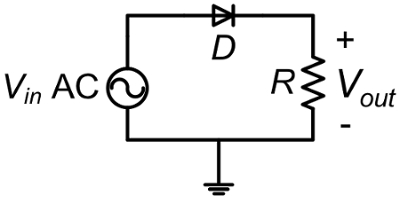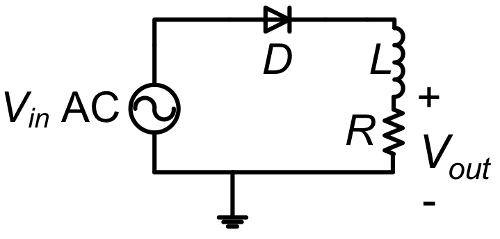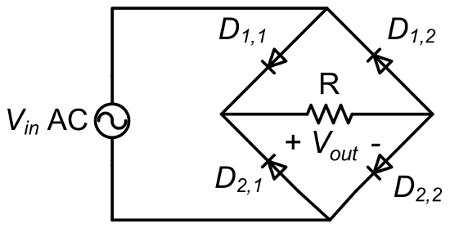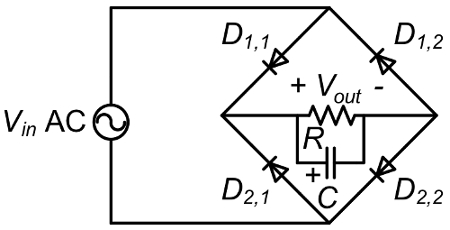Trial ends in
JoVE Science Education
Electrical Engineering

A subscription to JoVE is required to view this content.
You will only be able to see the first 20 seconds.

Single Phase Rectifiers
##### Science Education (Electrical Engineering)
AC Induction Motor Characterization
##### Science Education (Electrical Engineering)
Characterization of Magnetic Components

# Single Phase Rectifiers

### Overview

Source: Ali Bazzi, Department of Electrical Engineering, University of Connecticut, Storrs, CT.

A DC power supply is generally considered to be a device that supplies DC, or unidirectional, voltage and current. Batteries are one such power supply, however, they are limited in terms of lifetime and expense. An alternative method to providing unidirectional power is to transform AC line power to DC power using a rectifier.

A rectifier is a device that passes current in one direction, and blocks it in the other direction, enabling the transformation of AC to DC. Rectifiers are important in electronic circuits as they only allow current in a certain direction after a certain threshold forward voltage across them is overcome. A rectifier can be a diode, a silicon controller rectifier, or other types of silicon P-N junctions. Diodes have two terminals, the anode and the cathode, where current flows from anode to cathode. Rectifier circuits use one or more diodes that change AC voltages and currents, which are bipolar, to unipolar voltages and currents that can be easily filtered to achieve DC voltages and currents.

### Principles

Diode rectifiers are two-terminal semiconductor devices that pass current in one direction and block it in the other direction. Current passes from the anode to the cathode but not from the cathode to the anode. There is typically some leakage current in the blocking direction (cathode to anode), but it is very low. Diodes blocking current flow thus need to block a certain voltage level across from the cathode to the anode, so diodes are rated for their current carrying capability and their voltage blocking capability. When voltage across the diode terminals exceeds that voltage blocking rating, the diode operates in the breakdown region where it breaks and passes current both ways. The fact that diodes pass current in one direction leads to rectification capabilities where AC can be converted to DC.

Half-wave rectifiers (Fig.1 and Fig. 2) only pass half of the AC input voltage to the output while they block the negative half by providing a zero-output voltage. Full-wave rectifiers (Fig. 3 and Fig. 4) flip the polarity of the negative half to become positive in addition to passing the positive half. Though the outputs of these rectifiers are not smooth, they are by definition DC outputs, as the current flows in only one direction. However, these output waveforms are typically filtered in order to smooth the resulting output voltage.

The objective of this experiment is to study half-wave and full-wave single-phase rectifier operation for different load types. Rectification, along with the turn-off characteristics of diodes, are observed when the diode current reaches zero. Filtering the DC output voltage using an electrolytic capacitor is also studied.

### Procedure

ATTENTION: During this experiment, do not touch any part of the circuit while energized. The AC source is only grounded as shown in Fig. 1 and 2 when the function generator is a source. Do NOT ground the VARIAC.

1. AC Source Setup

For this experiment, two AC sources are used; a variable transformer (VARIAC) at a low frequency of 60 Hz and a function generator with 10 V peak sinusoidal output and 1 kHz frequency.

1. Before starting, connect the differential probe to one scope channel, and a regular probe to the other channel.
2. Adjust the buttons on the probes as follows: differential probe at 20X (or 1/20) and the regular probe at 10X. Do not forget to turn ON the differential probe.
3. On each channel's menu on the scope, set the probe to be at 10X. For the differential probe, manually multiply any measurements or results by two to reach the 20X desired.
4. To set up the function generator, make sure the 50 Ω OUTPUT is connected to a BNC-to-alligator cable.
1. Connect the alligator clips to a regular scope probe to observe the function generator output.
2. Set the output to be a sinusoid at 10 V peak and 1 kHz frequency with zero DC offset.
3. Observe the function generator output and adjust its settings to achieve the desired output waveform.
4. Once your signal is set, disconnect the BNC connector but keep the function generator ON to maintain its settings. Disconnect the scope probe from the generator output.
5. To setup the VARIAC, make sure the VARIAC output (looks like a regular receptacle) is not connected to any cable.
1. Keep the VARIAC OFF and make sure its knob is set to zero.
2. Slowly adjust the VARIAC knob to 5% output. This should yield around 10V peak voltage.

Half-Wave Rectifier

2. Resistive Load with High Frequency Input

1. Use the function generator as the AC source but keep it disconnected from the circuit for now.
2. On the proto board, build the circuit shown in Fig.1. The diode (D) is 2A01G-T rated for 50 V and 2 A while the load resistor (R) is 51 Ω.
1. Make sure the diode polarity is correct. The dash on the diode is at the cathode.
3. Before connecting the differential probe to the circuit, tie the probe's terminals together and adjust its measured waveform on the screen to show zero offset voltage.
1. Connect the differential voltage probe across the load resistor to observe the output voltage Vout.
2. Connect a regular probe across the AC side to observe the input voltage Vin.
3. Connect the function generator to the circuit.
4. Adjust the time base on the scope to show Vin and Vout for up to four fundamental cycles of Vin. Make a copy of the waveforms.
1. Zoom in at the diode turn-off region and make a copy of the waveforms.
5. Disconnect the function generator and remove the differential probe for load modifications. Keep the rest of the circuit and connections as they are.Figure 1: Half-wave rectifier with resistive load

3. Resistive-Inductive Load with High Frequency Input

1. Using the same circuit in Fig. 1, connect a 4.7 mH inductor (L) in series with the resistive load as shown in Fig. 2.
2. Connect the differential voltage probe across the load resistor to observe the resistor voltage Vout which has the same waveform shape as the R-L load current Iout.
3. Turn the function generator output ON.
4. Adjust the time base on the scope to show Vin and Vout for up to four fundamental cycles of Vin. Make a copy of the waveforms.
1. Zoom in at the diode turn-off region and observe the delay in the turn-off time. Make a copy of the waveforms.
2. Turn the function generator output OFF and disconnect it from the circuit.
3. Remove the inductor L and keep the rest of the circuit as it is.Figure 2: Half-wave rectifier with R-L load

4. Resistive Load with Low Frequency Input

1. Make sure the VARIAC output is at 5% and disconnected from the circuit. Connect the differential probe across the VARIAC, turn the VARIAC ON and slightly adjust its output to achieve 10 V peak.
1. Capture the waveform on the scope to use as your reference input voltage observation.
2. Turn the VARIAC OFF but do not change its voltage setting.
2. Using the same circuit from Fig. 1, i.e. with the inductor disconnected and the resistor being the only load, connect the VARIAC output using the plug-banana cable.
3. Connect the differential voltage probe across the load resistor to observe the output voltage, Vout.
4. Turn ON the VARIAC output. Stay away from the circuit and observe the waveforms on the scope. If you need to debug your circuit, power the VARIAC OFF first.
5. Adjust the time base on the scope to show Vout for up to four fundamental cycles. Make a copy of the waveform.
1. Zoom in at the diode turn-off region and make a copy of the waveforms.
6. Turn OFF the VARIAC and disassemble your circuit. Do NOT change the VARIAC voltage setting.

Full-Wave Rectifier

1. On the proto board, build the circuit shown in Fig. 3.
1. Make sure the diode polarity is correct. The dash on the diode is at the cathode.
2. Once the circuit is ready, connect the VARIAC output as the AC source.
3. Connect the differential voltage probe across the load resistor to observe the output voltage Vout.
4. Turn ON the VARIAC output. Stay away from the circuit and observe the waveforms on the scope. If you need to debug your circuit, power the VARIAC OFF first.
5. Adjust the time base on the scope to show Vout for up to four fundamental cycles of Vin. Make a copy of the waveforms.
1. Measure the peak-to-peak value of Vout by using the cursors.
6. Keep the probe connections as they are and Turn OFF the VARIAC and disassemble your circuit.
1. Do NOT change the VARIAC voltage setting.Figure 3. Full-wave rectifier with resistive load.

6. Resistive Load with Filtering Capacitor

1. Using the same circuit in Fig. 3, connect an electrolytic capacitor (C) in parallel with the resistive load as shown in Fig. 4.
1. Make sure the capacitor polarity is correct with the ( - ) terminal connected to negative side of the load.
2. Turn ON the VARIAC output. Stay away from the circuit and observe the waveforms on the scope. Before debugging the circuit, power the VARIAC OFF.
3. Adjust the time base on the scope to show Vout for up to four fundamental cycles of VIN. Make a copy of the waveforms.
1. Measure the peak-to-peak value of Vout by using the cursors and the AC coupling option for that channel (AC coupling eliminates the DC offset of a signal).
4. Return this to DC coupling once the measurement is made.
5. Turn OFF the VARIAC.
6. Disassemble the circuit and clean up the bench.Figure 4. Full-wave rectifier with resistive load and capacitive filtering

Single-phase rectifiers are used to convert AC supply voltage and current to DC, as required for powering equipment and digital electronic devices. Standard mains power supplied to homes and commerce is AC. However, most digital electronics are designed to run on DC power. Rectifiers are devices that can be used to transform AC electricity to a compatible DC supply. A rectifier passes current in only one direction, thereby transforming bipolar AC input to a unipolar rectified output. Rectifier circuits use one or more diodes to pass only positive or negative AC power, resulting in a pulsating source, which is then filtered to achieve smooth, consistent, DC voltage and current. This video introduces fundamental rectifier and diode circuitry concepts, demonstrates several common rectifier circuits, and tests the voltage output of rectifier circuits with variations in voltage input and loading configuration.

Rectifiers are devices used in electronic circuits to pass current in one direction and block it in the other direction. Rectifiers allow the passage of current only when a threshold forward voltage is surpassed. Diode rectifiers have two terminals, the anode and the cathode, with current flowing from anode to cathode and blocked from cathode to anode. Single-phase half-wave rectifiers pass voltage across across a single diode. In this circuit, only the positive half of the AC input voltage transmits to the output across the load resistor. If the diode were reversed, only the negative half of the AC input voltage would appear across the resistor. Voltage for the negative half of the AC cycle is blocked. With only one polarity, the RMS, or root mean squared, output voltage is reduced compared to that of the bi-polar input voltage. Full-wave rectifiers pass both half cycles of the AC input voltage across a four diode bridge circuit, as shown. Flipping the polarity of the negative half and yielding a higher average output voltage across the load resistor. Rectifiers result in a unidirectional, but pulsating current with the effect more apparent in half-wave rectifiers. However, the output of the rectifier is typically filtered by the addition of an inductor in series with the load resistance. In the full-wave rectifier, a capacitor assembled in parallel to the load resistor serves the same purpose. This video illustrates half and full-wave single phase rectifier operation with different output loads, diode turn off characteristics, and filtering of the DC output voltage using different circuits.

For this demonstration of rectifier operation, two different AC sources are used, high frequency, one kilohertz input, is produced using a function generator with 10 volt peak sinusoidal output. Low frequency 60 hertz input is supplied by a variac. Do not touch any part of the circuit while energized. When using the function generator source, the circuits are grounded as shown. Do not ground the variac supply. To set up the function generator for high frequency output, connect the differential probe to a oscilloscope channel one and a 10x probe to channel two. Adjust the scaling factors to 20x on the differential probe and 10x on the 10x probe. On the scope channel menus, set both probes to 10x. For the differential probe, manually multiply measurements by two to reach the 20x desired output. Next, connect a BNC to alligator cable to the 50 ohm output of the function generator and connect the alligator clips to the 10x scope probe. Set the output to a 10 volt peak and 1,000 hertz sinusoidal wave form with zero DC offset. Once the signal is set accordingly, disconnect the bnc connector and scope probe, but keep the function generator on to maintain its settings. To set up the variac for low frequency output, make sure that the output receptacle is disconnected, and that it is off with it's knob set to zero. Next, slowly adjust the variac knob to five percent output to achieve 10 volt peak.

First, test the half-wave rectifier with a high frequency input voltage and a resistive load. Build the circuit as shown, using a 51 ohm load resistor and a diode rated for 50 volts and two amps. Diode polarity is labeled with a dash symbol on the cathode end. Before connecting the differential probe to the circuit, connect the probe's terminals together and adjust the wave form to zero offset voltage. Then, connect the differential voltage probe across the load resistor to observe the output voltage and the 10x probe across the AC side to observe the input voltage. Next, adjust the time base on the scope to show input and output voltage for four cycles of input voltage. Disconnect the function generator and remove the differential probe from the circuit before making any modifications. Next, test the half-wave rectifier with high frequency input and a resistive inductive load. Reuse the circuit, adding an inductor in series with the resistor, as shown. As described previously, connect probes to the circuit and display wave forms of input and output voltage. Turn off the function generator, disconnect the differential probe, and remove the inductor from the circuit. Last, test the half-wave rectifier with low frequency input and a resistive load. Connect the differential probe across the variac and turn it on. Adjust the variac to obtain a 10 volt peak output, then turn the variac off without changing its voltage setting. Attach the variac output to the resistive circuit, as shown. Then, connect the differential voltage probe across the load resistor to observe the output voltage. Turn the variac on. Do not touch the circuit with the variac power connected and on. As described previously, display wave forms of input and output voltage.

First, test the full-wave rectifier with a resistive load. Build the circuit as shown, and connect the probes and the variac output to the circuit. As described previously, display wave forms of input and output voltage and measure the peak to peak voltages. Retaining the probe connections, turn off the variac and connect an electrolytic capacitor in parallel with the resistive load. Then observe the input and output voltage.

The first figure shows four cycles of an AC supply voltage and the output from a resistive load coupled to a half wave rectifier. Only the positive half cycle of the input AC voltage passes across the diode rectifier. If the input voltage of the half-wave rectifier circuit is sinusoidal, then the mean voltage output for a single diode with a resistive load is the input peak voltage divided by pi. When an inductor is added in series with the load resistor, the diode turn off region is delayed. This combination of inductor and resistor is a low pass filter. When the value of the inductor is sufficiently large, the oscillatory component of the output is blocked, leaving only the constant DC component. For a full-bridge rectifier, the input positive half cycles pass through the circuit and the negative half cycles are rectified to positive. Adding a sufficiently large capacitor filters out most of the voltage ripple, and provides the load with a consistent DC voltage.

Diode rectifiers are in most power supplies, chargers, variable frequency drives, and in many protection circuits. First, AC power adapters are used to convert power for DC supplied machines or to recharge DC batteries contained within devices. The adaptor can be as simple as a circuit consisting of a transformer to step down the voltage from the 120 volt wall supply, a four diode bridge full wave rectifier, and a capacitor to smooth the DC output voltage. Thyristors are silicone controlled rectifiers commonly used in light dimmers, motor speed controllers, and voltage regulators. By design, the thyristor is for alternating layers of P and N type semiconductors used to create an anode at the P type end, a cathode at the N type end, and a gate leap connected to the P type layer next to the cathode. Above a latching threshold, a current pulse into the gate switches the thyristor from off to on, allowing forward current flow from anode to cathode. This rectifies current flow in one direction and regulates output power with an integrated switching mechanism.

You've just watched JoVE's introduction to single-phase rectifiers. You should now understand how single-phase rectifiers work, common rectifier circuits and their output, and some common rectifier applications. Thanks for watching.

### Results

It is expected that a resistive load coupled to a half-wave rectifier will only see the positive half-cycle of the input AC voltage since the diode rectifier can pass current in one direction. With a full-bridge rectifier, the input positive and negative half-cycles are rectified to be positive, but adding a capacitor will filter out most of the voltage ripple and provide the load with a clean DC voltage.

When an inductor is added in series with the load, it is expected that the diode turn off will be delayed. This can be explained as follows: Diodes turn off under two conditions (that are required to coexist) 1) the current in the diode has to go to zero, and 2) the voltage across the diode (Anode-to-cathode voltage) is below the turn-on threshold. When an inductor is in series with the load, it stores energy and will act as a current source when the source is not available or is going negative at the diode's anode side. Therefore, inductor current will maintain the diode as forward biased until the inductor energy is dissipated. Essential equations that govern basic rectifier circuits with input Vin=V0cos(ωt):

Single diode and resistive load: <Vout>=V0/π  (1)

Diode bridge and resistive load: <Vout>=2V0/π  (2)

Diode bridge, current source load: <Vout>=2V0/π  (3)

### Applications and SummaryX